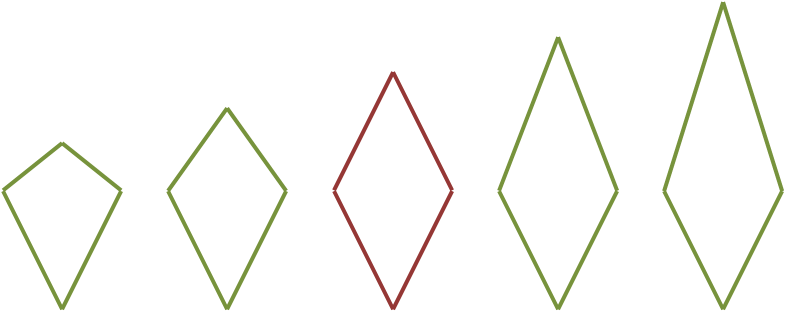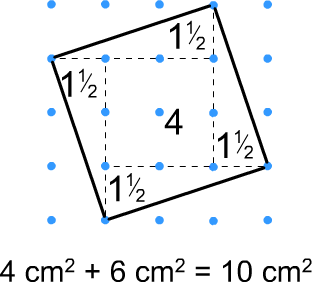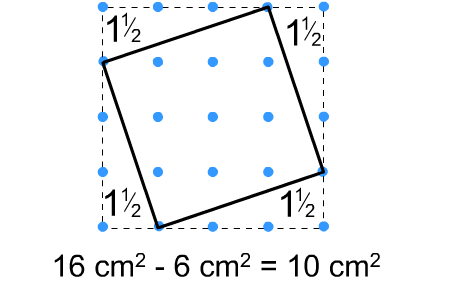#### You may also likeFour rods, two of length a and two of length b, are linked to form a kite. The linkage is moveable so that the angles change. What is the maximum area of the kite?### Making Rectangles, Making Squares

How many differently shaped rectangles can you build using these equilateral and isosceles triangles? Can you make a square?This gives a short summary of the properties and theorems of cyclic quadrilaterals and links to some practical examples to be found elsewhere on the site.

### Special Cases

How do you define a rectangle? There are lots of possible definitions, such as:

A quadrilateral with four right angles.

Does a square satisfy this definition?

This means that a square is a special type of rectangle: it meets all of the criteria for a rectangle but has other special properties. Take a look at these rectangles:One of these is special, and is given the extra name of a square, but it is still a rectangle. It is a special case of a rectangle.

Notice that all squares are rectangles, but not all rectangles are squares. Think about the right-hand rectangle above.

Here are some other examples of special cases:These are a collection of rhombuses, one of which is also a square.These are a collection of kites, one of which is also a rhombus.This is a collection of parallelograms, one of which is also a rectangle.

### Two standard methods for working out the areas of tilted squares, which may help to work out the areas of the quadrilaterals:

Splitting the tilted square into right-angled triangles and squares and adding the areas of the different parts:Boxing in the square, working out the area of the box and subtracting the areas of the unwanted right-angled triangles: